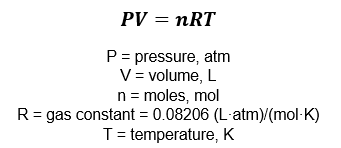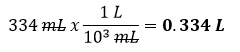# Problem: A 334-mL cylinder for use in chemistry lectures contains 5.257 g of helium at 23˚C.How many grams of helium must be released to reduce the pressure to 65 atm assuming ideal gas behavior?

###### FREE Expert Solution

Since the gas behaves ideally, the ideal gas equation can be used to solve this problem:Calculate the number of moles of gas required for P = 65 atm:

Given:

V = 334 mLT = 23° C + 273.15 K = 296.15 K

82% (469 ratings)###### Problem Details

A 334-mL cylinder for use in chemistry lectures contains 5.257 g of helium at 23˚C.

How many grams of helium must be released to reduce the pressure to 65 atm assuming ideal gas behavior?